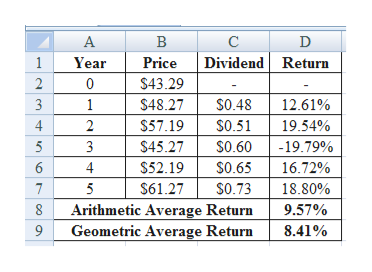# A stock has had the following year-end prices and dividends: Year Price Dividend0 \$43.29  - 1  48.27 \$.48 2  57.19  .51 3  45.27  .60 4  52.19  .65 5  61.27  .73   What are the arithmetic and geometric average returns for the stock? (Do not round intermediate calculations. Enter your answers as a percent rounded to 2 decimal places, e.g., 32.16.)   Arithmetic average return %Geometric average return %

Question
69 views

A stock has had the following year-end prices and dividends:

 Year Price Dividend 0 \$ 43.29 - 1 48.27 \$ .48 2 57.19 .51 3 45.27 .60 4 52.19 .65 5 61.27 .73

What are the arithmetic and geometric average returns for the stock? (Do not round intermediate calculations. Enter your answers as a percent rounded to 2 decimal places, e.g., 32.16.)

 Arithmetic average return % Geometric average return %
check_circle

Step 1

Calculation of Arithmetic and Geometric Average Return:

The arithmetic average return is 9.57% and geometric average return is 8.41%.

...help_outlineImage TranscriptioncloseА В C Dividend Return 1 Year Price \$43.29 0 \$0.48 1 \$48.27 12.61% \$0.51 4 \$57.19 19.54% \$45.27 5 3 \$0.60 -19.79% \$52.19 \$0.65 6 4 16.72% \$0.73 7 5 \$61.27 18.80% Arithmetic Average Return 8 9.57% Geometric Average Return 8.41% n CO O fullscreen

### Want to see the full answer?

See Solution

#### Want to see this answer and more?

Solutions are written by subject experts who are available 24/7. Questions are typically answered within 1 hour.*

See Solution
*Response times may vary by subject and question.
Tagged in